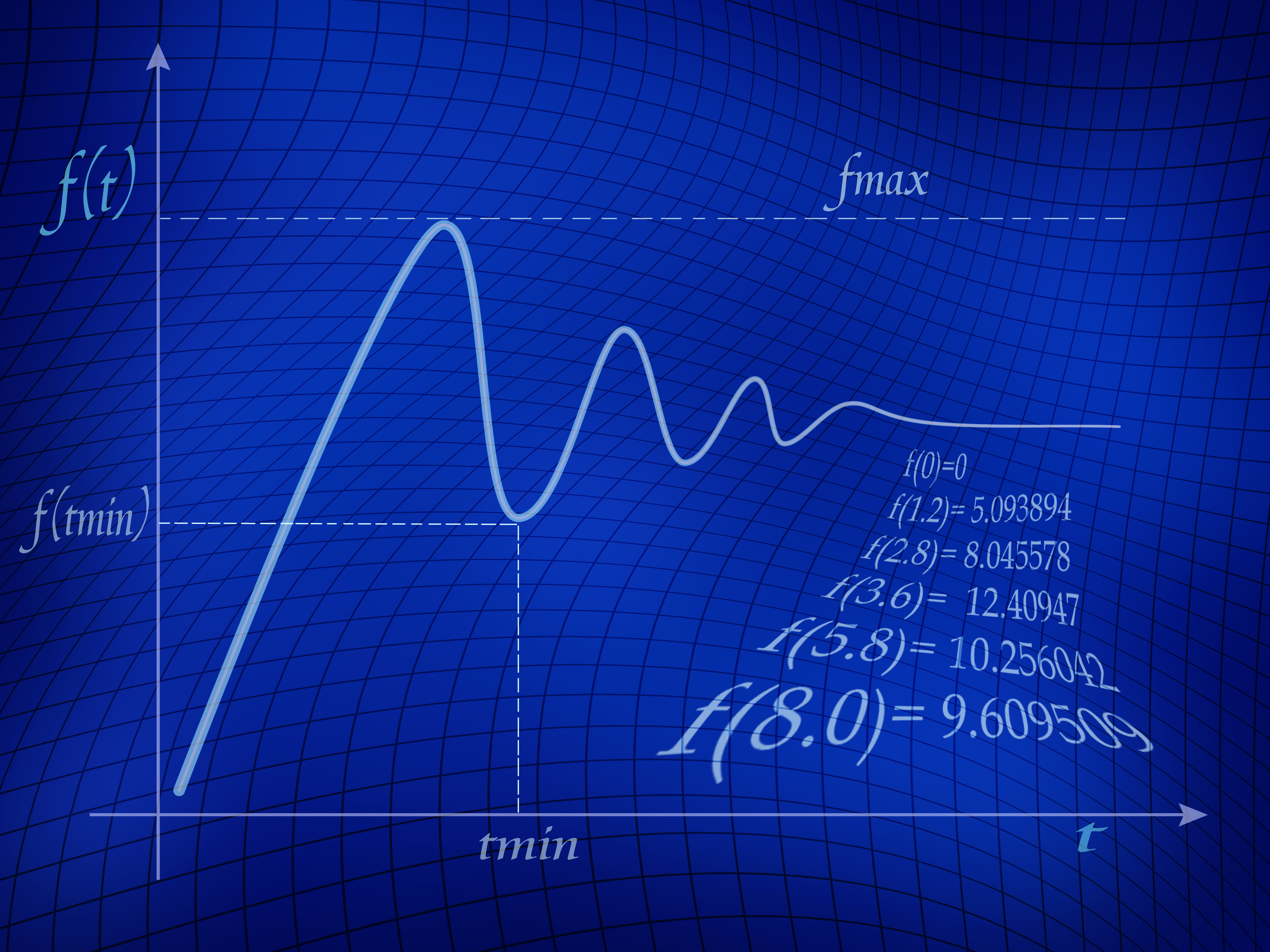### Mathematical OptimizationIn optimization, one characterizes values of decision variables in order to satisfy an objective subject to a given set of constraints. In mathematical optimization, the objective and constraints are given as models of real-world phenomena. This means that they may be given algebraically or as outputs of a computer simulation.

Throughout the rapid expansion of the field of mathematical optimization over the past half century, it has been an extremely powerful tool in Industrial and Systems Engineering. It provides comprehensive and elegant methodologies to support decision making where goals such as cost, efficiency, profit, or risk are optimized under limited resources or other constraints.

Optimization problems often exhibit rich structures that can beleveraged when one seeks solutions or characterizations thereof. These different structures can also be exploited in the development of specialized algorithms for solving increasing larger and more complicated problems. At Lehigh ISE, we investigate a wide spectrum of challenging optimization problems for which we also develop, analyze, and implement efficient and reliable algorithms. We perform cutting-edge research spanning all flavors of optimization problems:  convex, conic, integer, combinatorial, black-box/derivative-free, nonconvex, nonsmooth, robust, stochastic, among others.

###### Lehigh's research groups / institutes:

ISE Computational Optimization Research at Lehigh (COR@L)###### Illustrative publications by Lehigh's ISE faculty:

J. Peng, C. Roos, and T. Terlaky, Self-regularity: A New Paradigm for Primal-Dual Interior-Point Algorithms, Princeton Series in Applied Mathematics, Princeton University Press, Princeton, 2002.